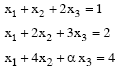# GATE | GATE CS 2008 | Question 85

The following system of equationshas a unique solution. The only possible value(s) for α is/are
(A) 0
(B) either 0 or 1
(C) one of 0, 1 or -1
(D) any real number
(E) any real number other than 5

Explanation: The choice E was not there in GATE paper. We have added it as the given 4 choices don’t seem correct.

```Augment the given matrix as

1 1 2 | 1
1 2 3 | 2
1 4 a | 4

Apply R2 <- R2 - R1 and R3 <- R3 - R1

1 1 2 | 1
0 1 1 | 1
0 3 a-2 | 3

Apply R3 <- R3 - 3R2

1 1 2 | 1
0 1 1 | 1
0 0 a-5 | 0

So for the system of equations to have a unique solution,

a - 5 != 0
or

a != 5
or
a = R - {5} ```

Thanks to Anubhav Gupta for providing above explanation.

Readers can refer below MIT video lecture for linear algebra.